# Difference between revisions of "Testpage1"

Jump to: navigation, search

Tidal wave deformation and tidal bores

Table 1. Estuaries and rivers with substantial tidal bores (bore height of half a meter up to several meters).
Estuary/river name Maximum tidal range [m] Tide gauge location Country
Shannon River 5.6 Shannon Ireland
Humber,Trent 8.3 Hull UK
Great Ouse 7.4 King's Lynn
Severn 12 Portishead
Dee 9.8 Flint
Mersey 10 Liverpool
Ribble 10.2 Lytham
Morecambe bay, Nith River, River Kent 10.9 Morecambe
River Eden 10.3 Silloth
Seine 8.5 Honfleur France
Canal de Carentan 7 Carentan
Baie du Mont Saint Michel – Sélune River 14 Granville
Garonne, Dordogne 6 Bordeaux
Pungue 7 Beira Mozambique
Qiantang 5 Ganpu China
Indus 4 Port Muhammad Bin Qasim Pakistan
Hooghly 5.7 Sagar Island India
Brahmaputra 5.7 Chittagong Bangladesh
Sittaung 6.3 Moulmein Myanmar
Batang Lupar River 5.6 Kuching Sarawak
Kampar River 5.3 Pulo Muda Sumatra
Hooghly 5.7 Sagar Island India
Fly , Bamu, Turamu Rivers 4.2 estuary West Papua
Styx 6.4 Mackay, Queensland Australia
Daly River 7.9 estuary
Turnagain Arm, Knick Arm 7.9 Anchorage Alaska
Bay of Fundy, Petitcodiac and Salmon rivers 16 Truro Canada
Colorado River 7.7 San Filipe Mexico
Amazon, Araguira, Guama, Capim and Mearim Rivers 3.4 Macapa Brazil

## Tidal wave deformation in shallow water

Tides result from the response of the ocean water bodies to the attractive gravitational forces by sun and moon. Tidal motion in the oceans can be described by a limited number of sinusoidal components, because earth's rotation and the relative movements of sun and moon have a cyclical character, see the article Ocean and shelf tides. The semidiurnal lunar tide (M2) is usually the dominant component, in which case the ocean tide can be described fairly accurately with a single sine function.

However, it is well known that this simple description is not valid for shallow coastal areas. The tidal wave is distorted as it propagates from the ocean to coastal areas where the tidal amplitude is no longer negligibly small compared to the average water depth. Negligibly small means: a tidal amplitude that is no greater than a few percent of the water depth. In some coastal areas, particularly in estuaries and tidal lagoons, the tidal amplitude is just a few times smaller than the average water depth or even of similar magnitude.

In shallow coastal areas, the distortion of the tide can be so strong that the durations of rising tide and falling tide become very different and that a large difference arises between the peak flow velocities of flood and ebb. Often the duration of rising tide is much shorter than the duration of falling tide. In the most extreme case, the duration of tidal rise becomes so short that a hydraulic jump develops at the front of the tidal wave. The front of the tidal wave appears as a propagating wall of water, a so-called tidal bore (French: mascaret, Spanish: macareo, Brazilian: pororoca).

## Tidal wave deformation in the absence of friction

The distortion of the tide described above is related to a difference in propagation speed of the high-water crest of the tidal wave and the low-water trough. This difference already occurs when the water depth below the wave crest is slightly greater than the water depth below the wave trough. This can be easily demonstrated for a tidal wave that propagates in $x$-direction with time $t$ in deep water with little loss of friction. The tidal equations (momentum balance and mass balance) can in this case be simplified to

$\Large\frac{\partial u}{\partial t}\normalsize + u \Large \frac{\partial u}{\partial x}\normalsize + g \Large \frac{\partial \zeta}{\partial x}\normalsize =0 , \quad \quad (1)$

$\Large \frac{\partial \zeta}{\partial t}\normalsize + h \Large \frac{\partial u}{\partial x}\normalsize + \Large \frac{\partial u \zeta }{\partial x}\normalsize =0 , \quad \quad (2)$

where $\zeta$ is the tidal wave surface elevation, $u$ is tidal velocity, $h$ is the mean water depth and $g$ the gravitational acceleration. The equations are linear except for the second term in Eq. (1) and the last term in Eq. (2). These non-linear terms are much smaller in the present case than the linear terms.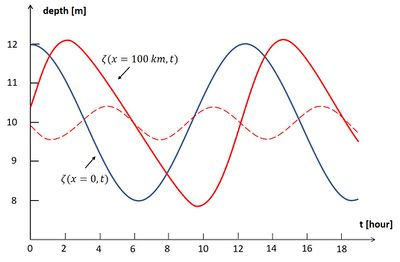Figure 1: Deformation of a frictionless propagating wave, corresponding to Eqs. (1) and (2). The blue curve is the sinusoidal tidal wave at $\small x=0, \zeta(x=0,t) = a \cos \omega t , \; a = 1 m$. The red curve is the distorted tidal wave after travelling a distance of $\small x=$ 100 km without friction in a channel of 10 m mean depth, $\small \zeta(x,t) = \zeta^{(1)} + \zeta^{(2)}$, with $\small \zeta^{(1)} = a \cos(\omega t - kx) , \; \zeta^{(2)} = \frac{3 a^2}{4h} kx \sin(2 \omega t – 2kx)$. The dotted red line is $\small \zeta^{(2)}$. The red curve displays a shorter tidal rise and a longer tidal fall: the tidal wave crest has propagated faster than the tidal wave trough.

The equations can therefore be solved to a first approximation by substituting the solution $\zeta^{(1)}$ of the linear equations into the nonlinear terms. The solution of the resulting linearized equations introduces a small M4 tidal component $\zeta^{(2)}$ with twice the M2-tidal frequency. This M4 component modifies the duration of rising water and falling water, because the propagation speed $c^+$ of the high-water wave crest is higher than the propagation speed $c^-$ of the low-water wave trough:

$c^{\pm}=(1 \pm \Large \frac{3a}{2h}\normalsize) \; \sqrt{gh} , \quad \quad(3)$

where $a$ is the amplitude of the M2 tide. See Fig. 1; a derivation is given in the appendix. This equation suggests that after some time the high-water wave crest overtakes the low-water wave trough. However, this can only happen when the amplitude of the M4 is of the same order of magnitude as the M2 tide, which violates the assumption underlying the linearization of the tidal equations.

The approximate validity of the result (3) is restricted to the zone where M4 is much smaller than M2. In spite of the restricted validity of Eq. (3) to large water depths and small tidal amplitude, the following general rule remains valid: the difference in propagation speed between high water and low water increases when the ratio $a/h$ increases. Numerical simulations with fully nonlinear mathematical models show that tidal distortion, with decreasing tidal rise period, occurs when the value of the parameter $a/h$ remains sufficiently large when the tidal wave propagates over a shallow seabed . In this case, the tidal wave transforms into a tidal bore when the propagation distance over the shallow seabed is sufficiently large. Not only tidal waves, but any long wave (characterized by a wavelength much larger than water depth) may transform into a bore when propagating over a shallow bed. Examples are: wave uprush on a beach (see the article Swash zone dynamics) and runup of a tsunami wave on a sloping bottom .

## Tidal wave deformation in the presence of friction and intertidal areas

The formation of a tidal bore is restricted by the condition that a sufficiently large ratio $a/h$ is maintained when the tidal wave travels over a shallow bed. In many cases this condition is not fulfilled, because the tidal amplitude decreases during propagation. The two main reasons for decrease of the tidal amplitude are: (1) tidal wave damping by friction and (2) lateral spreading of the flood tidal wave. These two nonlinear processes also influence the propagation of the high-water wave crest and the low-water wave trough. This can be demonstrated in the same way as previously, assuming that the nonlinear terms in the equations are much smaller than the linear terms.

We consider a straight prismatic tidal channel with mean depth $h$ , channel width $b_C$ and lateral flood storage zones of width $b_S= b_{S0} \; ( 1 + \zeta / a)$, where $\zeta / a$ is the ratio of tidal surface elevation and tidal amplitude, see Fig.2 . When frictional effects are much stronger than inertial effects, the one-dimensional depth-averaged tidal equations for small values of $a/h$ can be simplified to

$g \Large \frac{\partial \zeta}{\partial x}\normalsize + r \Large \frac{u}{h+\zeta}\normalsize \approx g\Large \frac{\partial \zeta}{\partial x}\normalsize + r \Large \frac{u}{h}\normalsize – r \Large \frac{u \zeta}{h^2}\normalsize =0 , \quad \quad (4)$

$(b_C+ b_S) \Large \frac{\partial \zeta}{\partial t} \normalsize + \Large \frac{\partial (u b_C(h+\zeta)) }{\partial x} \normalsize = (b_C+ b_{S0}) \Large \frac{\partial \zeta}{\partial t} \normalsize + b_{S0} \Large \frac{\zeta}{a} \frac{\partial \zeta}{\partial t} \normalsize + h b_C \Large \frac{\partial u}{\partial x}\normalsize + b_C \Large \frac{\partial (\zeta u)}{\partial x}\normalsize =0 . \quad \quad (5)$

The friction term in Eq. (4) has been linearized; $r$ is the corresponding friction coefficient (dimension [m/s]), which has a value that typically ranges between 0.002 - 0.004 m/s. We assume $b_S \lt \lt b_C$, and because $a/h \lt \lt 1$ the nonlinear terms in these equations are much smaller than the linear terms. A first order solution $\zeta^{(1)}$ can be found by substituting the solution of the linear equations in the nonlinear terms. The nonlinear terms then generate a small M4 tidal component $\zeta^{(2)}$, which affects the duration of tidal rise and tidal fall. This is because the propagation speed $c^+$ of the high-water wave crest differs from the propagation speed $c^-$ of the low-water wave trough (see the appendix):

$c^{\pm} \approx [1 \pm (2 - \sqrt{2}) \Large\frac{a}{h}\normalsize \mp \Large\frac{b_{S0}}{2b_C}\normalsize ] \; \sqrt{gh} \; \sqrt{ \Large\frac{2 \omega h}{r} \frac{b_C}{b_C +b_{S0}}\normalsize} , \quad \quad (6)$

where $\omega$ is the M2 radial frequency. This expression shows that the tidal propagation speed is reduced for increasing values of the nondimensional friction coefficient $\Large \frac{r}{h \omega}$. As in the previous example, the high-water (HW) propagation speed $c^+$ increases and the low-water (LW) propagation speed decreases with increasing values of $a/h$. The propagation of the LW trough of the tidal wave is more delayed than the propagation of the HW crest, due to stronger friction at smaller water depths. The period during which the tide rises is thus shortened with respect to the duration of falling tide. However, the opposite occurs for increasing values of the relative flood storage width $b_{S0}/b_C$. The HW propagation speed $c^+$ decreases relative to the LW propagation and the period during which the tide rises is thus lengthened with respect to the duration of falling tide. This can be understood by noting that the lateral expansion of the tidal flood wave over the intertidal area reduces its propagation speed and thus delays the time of HW in the up-channel zone. Hence, shallowness of the channel (large $a/h$) and extension of the intertidal area (large $b_{S0}/b_C$) have counteracting effects on tidal wave distortion.

## Tidal wave deformation in a converging channel

Friction not only slows down tidal propagation; friction also dampens the tidal amplitude. As shown above, a tidal bore only occurs when the tide propagates into a shallow zone where the relative tidal amplitude $a/h$ is sufficiently large. In such shallow areas, however, strong friction dampens the tidal amplitude and thus reduces the ratio $a/h$. This reduction is even more important in estuaries with large intertidal areas, because the tidal amplitude decreases further when tidal flood waters spill over the intertidal areas. However, the opposite occurs when the tide propagates into a tidal channel that becomes progressively narrower in up-channel direction, see Fig. 3. Instead of expanding laterally, the tidal wave is contracted when propagating. In the hypothetical case of no friction, conservation of the tidal energy flux along the channel requires up-channel amplification of the tidal amplitude. This follows directly from the tide propagation equations in a channel of exponentially converging width $b=b_0 \; e^{-x/L_b}$. The linearized tidal equations for a channel with uniform depth $h$ read:

$\Large\frac{\partial u}{\partial t}\normalsize + g \Large \frac{\partial \zeta}{\partial x}\normalsize + r \Large \frac{u}{h}\normalsize = 0 , \quad \quad (7)$

$b \Large \frac{\partial \zeta}{\partial t}\normalsize + \Large \frac{\partial (bhu)}{\partial x}\normalsize = b [ \Large \frac{\partial \zeta}{\partial t}\normalsize + h \Large \frac{\partial u}{\partial x}\normalsize – u \Large \frac{h}{L_b} \normalsize ] = 0 . \quad \quad (8)$

Solving these linear equations (only tide, no river discharge) yields

$\zeta = a e^{-\mu x} \; cos(kx-\omega t) \quad$ with $\quad 2 L_b \mu = \normalsize -1 + \Large[\normalsize 1 – (\Large \frac{1}{2} \normalsize + 2 K_0^2) +\large[\normalsize (\Large \frac{1}{2}\normalsize + 2 K_0^2 )^2+ 4 (K_c^2 - K_0^2) \large]^{\large 1/2} \Large]^{\large 1/2} \normalsize, \quad \quad (9)$

where $K_0 = \Large \frac{\omega L_b}{\sqrt{gh}}\normalsize , \; K_c = \Large \frac{r \omega L_b^2}{g h^2} \normalsize$. The damping factor $\mu$ is positive for large friction and large convergence length ($K_c \gt K_0$). However, for small friction and small convergence length ($K_c \lt K_0$) the damping factor is negative: the tide is amplified when propagating up-channel. Even in the case of strong friction, the tide is only slightly damped or even amplified if the convergence length $L_b$ is sufficiently small. Large tidal bores can therefore develop in strongly converging estuaries if the relative tidal amplitude $a/h$ is sufficiently large .

The solution of the Eqs. (7) and (8) also yields an expression for the wave propagation velocity $c$:

$c = \Large \frac{\omega}{k}\normalsize = 2 \omega L_b \Large[\normalsize - 1 + (\Large \frac{1}{2} \normalsize + 2 K_0^2) +\large[\normalsize (\Large \frac{1}{2}\normalsize + 2 K_0^2 )^2+ 4 [K_c^2 - K_0^2] \large]^{\large 1/2} \Large]^{\large -1/2}\normalsize . \quad \quad (10)$

The expressions (9) and (10) show that tidal wave propagation depends on only two parameters, $K_0$ and $K_c$. The linear equations (7) and (8) do not describe tidal wave deformation; for this, nonlinear terms have to be included ($u \partial u /\partial x$ and time varying water depth $h+\zeta$ in Eq. (7) and $b \zeta \partial u / \partial x$ in Eq. (8)). For weak nonlinearity these equations can be solved analytically; the solution involves the additional small parameter $a/h$. Tidal wave deformation in an exponentially convergent estuary with uniform depth is entirely determined by three parameters: $K_0, K_c, a/h$ . If intertidal areas $b_S$ and river discharge $Q_R$ are included, two additional parameters $b_{S0}/b_C$ and $Q_R/(a b_C L_b \omega)$ are needed to characterize tidal wave deformation. It should be borne in mind that although many estuaries have an upstream converging width, the assumption of exponential width convergence is for most estuaries a very rough approximation. Often only a limited part of the estuary can be represented in this way.

An example of tidal wave deformation and tidal bore formation is shown in Figs. 4 and 5 for the converging Seine estuary.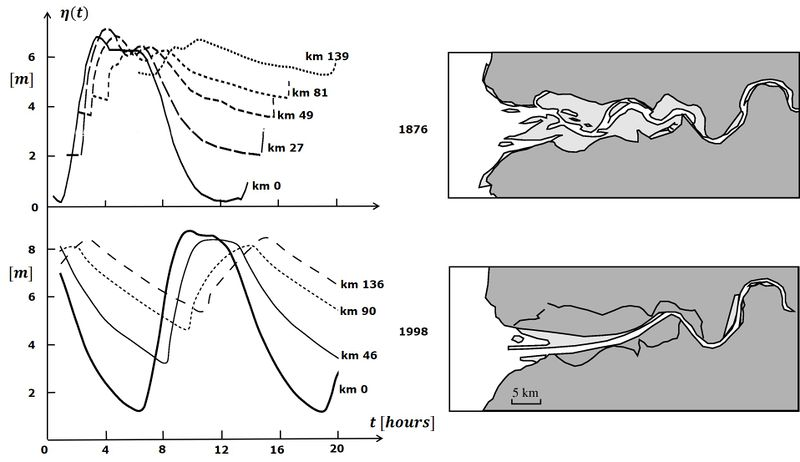Figure 4: Tidal wave distortion and tidal bore formation in the Seine estuary. The figures at the left show simultaneously recorded tide curves during springtide, for locations at various distances from the estuarine mouth. The images on the right show the morphology of the Seine estuary. The upper figures relate to the situation in the 19th century, when the morphology of the Seine estuary was almost in a natural state, with large shoals at the mouth (mouth bars) and an inner system with multiple channels and extensive intertidal areas. The 1876 tide curves (Comoy, 1881 ) display strong damping and delay in tidal propagation, especially for the low-waters. Tidal propagation over this complex shallow geometry resulted in a tidal bore that reached its largest amplitude at about 50 km from the mouth. The lower panels relate to the current situation. In the course of the 20th century, and especially in the period 1970-1980, the morphology of the estuary was greatly changed by artificial interventions. The estuarine main channel was deepened, especially in the mouth zone, and fixed by submerged dikes. Large parts of the intertidal areas were diked and filled with dredged materials. Tidal damping and tidal distortion were greatly reduced. At present a small tidal bore occurs only under extreme tides and further inland than in the past.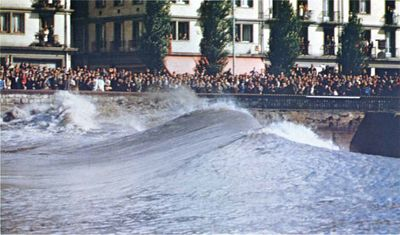Figure 5: Picture taken in 1963 at springtide of the tidal bore in the Seine at Caudebec (about 50 km upstream from the estuarine mouth).

## Tidal bore formation in rivers

In the foregoing, processes have been analyzed that lead to distortion of the tidal wave - possibly resulting in creating a tidal bore - for situations with a uniform channel depth. The influence of depth differences has not been taken into consideration. However, in practice depth differences can play a role. The tidal bore often occurs in a shallow river that discharges into the estuary from the higher upstream zone. The tidal bore develops when the tidal wave surges into the shallow river. For this it is necessary that the tidal wave already acquires strong asymmetry when propagating through the estuary. This process, as pictured schematically in Fig. 6, bears some resemblance to the uprush of a long swell wave or a tsunami wave on the beach.Figure 6: Schematic representation of the development of a tidal bore when the distorted tidal wave surges into an upstream shallow tidal river . This is also similar to the situation where a fast rising tide spills over a tidal flat at the end of the ebb period.

River flow and river bed slope both influence tidal bore formation. River flow contributes to tidal wave deformation by enhancing the longitudinal velocity gradient and river bed slope by upstream reduction of the water depth. However, river flow and river bed slope also contribute to tidal wave damping and thus oppose tidal bore formation. The latter effect usually dominates. Observations show that the tidal bore in the Garonne and Dordogne (France) is suppressed at high river runoff. The opposite occurs in the Guamá-Capim river system (Amazon tributaries), where tidal bores are observed only at high river discharges (in conjunction with high equinoctial tides).

## Sediment transport and estuarine morphology

In a converging (funnel-shaped) estuary with strong friction the tidal velocity $u$ is mainly determined by the water surface slope $\partial \zeta /\partial x$, according to Eq. 4. A short period of tidal rise compared to the period of tidal fall implies steeper water surface slopes during flood than during ebb. Hence, maximum flood velocities are higher than maximum ebb velocities. Because the transported sediment load increases more than linearly with the current velocity (see the articles Sand transport and Sediment transport formulas for the coastal environment), sediment fluxes during flood tide are higher than sediment fluxes during ebb tide. Flood-dominant tidal asymmetry thus produces a net import of sediment into the estuary. It can therefore be expected that estuaries become progressively shallower and narrower. Several processes prevent complete siltation of estuaries: (1) river outflow, (2) Stokes transport (water outflow compensating for the net influx due to greater mean water depth during flood than during ebb) and (3) reduction of tidal asymmetry due to the development of intertidal areas. One may thus conclude that the natural morphology of an estuary is largely due to self-organizing processes (see also the article Morphology of estuaries). This holds in particular for estuaries in a sedimentary environment without geological constraints. Funnel shape, sand bars and meandering channels are typical characteristics of self-organized estuaries, see also the article Morphology of estuaries.

## Occurrence of tidal bores

Table 1 gives an overview of estuaries and tidal rivers in which significant tidal bores have been observed. It can be concluded from the table that tidal bores often occur in megatidal zones - areas where the maximum tidal range is greater than 6 m. However, it appears that substantial tidal bores can also occur in areas where the tidal range is smaller, although this is more the exception than the rule. This exception seems to be limited to tropical and subtropical countries. Rivers are generally shallower in these regions due to abundant supply of fine sediments. Another possible reason is the relative importance of K1 and O2 diurnal tidal components, which can combine with the semidiurnal M2 tide to yield persistent tidal asymmetry  (see also the article Ocean and shelf tides). However, for the rivers listed in Table 1 this does not seem to play an important role .

Engineering works (weirs, dredging) during the past century have weakened or even completely suppressed tidal bores in many rivers, for example in the rivers Seine, Loire, Charente and Petitcodiac.

## Characteristics of tidal boresFigure 7: Schematic representation of a hydraulic jump propagating with velocity $c$ in a moving frame. In this frame moving with velocity $c$ the hydraulic jump appears stationary; time derivatives are zero. The corresponding mass and momentum balance equations from which the propagation characteristics can be derived are indicated in the figure. The symbols used stand for: $u$= flow velocity, $h$= water depth, $g$=gravitational acceleration; subscripts 1 and 2 indicate upstream and downstream conditions, respectively.

In the previous sections the processes that lead to the initiation of tidal bores have been discussed. Nonlinear processes are becoming increasingly important in the further development of tidal bores. These processes can no longer be analyzed with analytical methods, but only with numerical simulation models. In the final stages of development - the emergence of a breaking tidal wave - even the long-wave equations (Eqs. 1,2; 4,5; 7,8) are no longer applicable, but non-hydrostatic effects must be taken into consideration. This will be discussed further in the next section.

Once the tidal bore has fully developed as a hydraulic jump, its propagation characteristics can be derived form the mass and momentum balance equations, see Fig. 7. By eliminating $c-u_1$ or $c-u_2$ from these equations two equivalent expressions can be derived for the height of the tidal bore:

$\Delta = \Large \frac{h_1}{2} [\normalsize 3 - \sqrt{1 + 8 F_1^2} \Large ] \normalsize = \Large \frac{h_2}{2} [\normalsize - 3 + \sqrt{1 + 8 F_2^2} \Large ] \normalsize, \quad \quad (11)$

where $\Delta=h_1 -h_2$ is the bore height and the Froude numbers are defined as

$F_{1,2} = \sqrt{(u_{1,2}-c)^2 / g h_{1,2}}$.

For $\Delta \lt 0.4 h_1$ the tidal bore propagation speed $c$ can be fairly well represented by

$c \approx \Large \frac{1}{2} \normalsize (c_1 + c_2) , \quad \quad (12)$

where $c_{1,2} = u_{1,2} + \sqrt{g h_{1,2}}$.

If the bore velocity $c$ is equal to $c_1$ (i.e. $F_1 = 1$) the bore height $\Delta$ is zero. Another requirement for a tidal bore is therefore $c \lt c_1$ or $F_1 \lt 1$. Because $c_1$ is the propagation speed of disturbances upstream of the bore, these disturbances (undulations) will catch up to the bore. A slowly propagating bore ($F_1 \lt \lt 1$) will thus grow faster and become higher than a fast propagating bore ($F_1$ close to 1).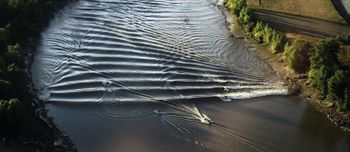Figure 8. Non-breaking undular tidal bore at Podensac in the Garonne River at high spring tide (tidal range 6 m at Bordeaux) and low river runoff (128 m3/s). The height of the lead wave is 1-1.5 m and the propagation speed about 15 m/s . The wavelength of the tidal bore undulations (whelps) in the midchannel is strongly reduced due to the interaction with the gently sloping river banks.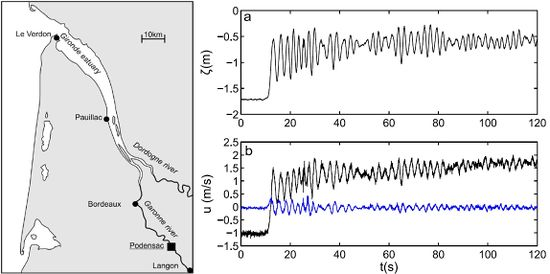Figure 9. Observations of surface elevation and current velocity at the passage of an non-breaking undular bore with Froude number $\small F_2=1.24$ in the Garonne River at Podensac, for a large spring tidal range and low river discharge. (a) Surface elevation $\small \zeta$ with respect to French datum; (b) Velocity components measured at 1.2 m above the river bed (along-channel component in black and vertical component in blue). At the wave front passage, the along- channel velocity increases abruptly from -1 to 1.4 m/s in 2.5 s. The whelps are associated with significant vertical motion with vertical velocity ranging from -0.25 to 0.25 m/s. The along-channel velocity and the elevation signals are in phase. The secondary wavefield, of period 2.5–3 s, is characterized by well-defined wave groups with a period of about 40 s .

Different types of tidal bores can be distinguished. From Eq. (11) it follows that tidal bores only occur if the Froude number $F_2$ is greater than 1. If $F_2$ is just above 1, the tidal bore has an undulating character; an example is the tidal bore in the Garonne river, see Fig. 8. The corresponding temporal variation in surface elevation and current velocity at the passage of the tidal bore, measured at a fixed station, is shown in Fig. 9. Non-breaking undular bores gradually fade away during up-river propagation, by energy loss at the wave front. This is mainly due to upstream energy radiation by the secondary wavefield.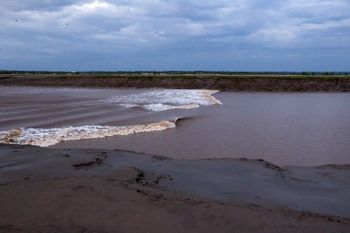Figure 10. Breaking undular tidal bore in the Petitcodiac River at Moncton, Canada. Photo credit: Jim Hoffman

When the Froude number is greater than approximately 1.3, the leading wave starts breaking. The undular breaking bore is illustrated in Fig. 10 for the Petitcodiac River. It is the most common type of tidal bores. It corresponds to a bore height of approximately $\Delta \approx 0.5 h_2$.

With even larger Froude numbers, approximately from $F_2 \gt 1.6$  (bore height $\Delta \approx h_2$ or more), undular perturbations catch up to the tidal bore, which takes the shape of a hydraulic jump. An example is the tidal bore in the Kampar River, Fig. 11. The breaking tidal bore is highly turbulent, forming a roller with massive entrainment of air bubbles. It exerts a strong shear stress on the bed and brings high quantities of sediment into suspension that are transported upstream by the tidal current. Strong sediment resuspension at the passage of a tidal bore is illustrated by field measurements in the Baie du Mont Saint Michel, shown in Fig. 12 (after Migniot, 1998 ). Similar strong bed erosion and sediment resuspension at the passage of a tidal bore were observed at field measurements in the Garonne River. The energy dissipated at the breaking bore front causes its gradual decay when propagating upstream.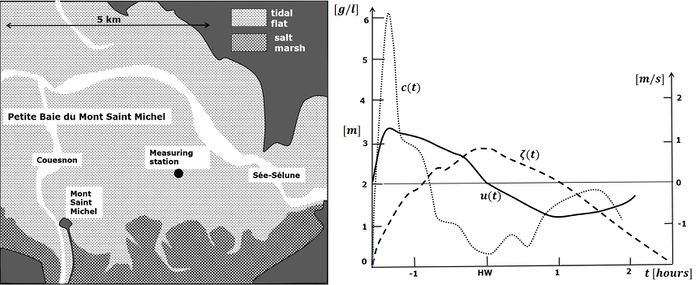Figure 12. Tide elevation $\small \zeta$ (above the dry bed), current velocity $\small u$ and suspended sediment concentration $\small c$ during springtide on a tidal flat in the Baie du Mont Saint Michel. The measurements started when the tidal flat at the measuring station was (almost) dry, just before the tidal bore spilled over the tidal flat. A rapid rise of the current velocity was recorded when the bore arrived. The suspended sediment concentration (mainly fine silty sand) peaked sharply at the passage of the bore front and quickly felt down afterwards. During the remaining flood period and during ebb the suspended sediment concentrations were much lower. The measurements illustrate the strong bottom stirring and sediment resuspension produced by the turbulent bore front, which significantly contributes to landward sediment transport and tidal basin infill .

## Modelling of tidal bores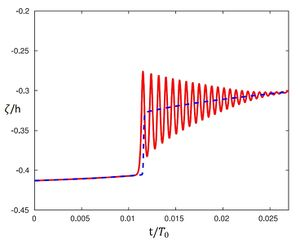Figure 13. Model simulation of an undular tidal bore passing at a fixed station. Red curve: Fully nonlinear Boussinesq-type model. Blue dotted curve: Non-Linear Shallow-Water model. The model simulation is carried out for an idealized geometry similar to the Garonne River, see Fig. 9 . Symbols: $\small \zeta$= water surface elevation with respect to French datum, $\small h$= mean channel depth, $\small t$= time, $\small T_0$= tidal period.

The linear long-wave equations (1,2), (4,5) and (7,8) do not represent the process of bore formation, and neither do the weakly non-linear extensions, which assume small tidal amplitude ($a/h \lt \lt 1$) and large wavelength, $\lambda \gt \gt h$. These assumptions are not valid for undular tidal bores; non-hydrostatic dispersive effects must be taken into account. Solutions to the general nonlinear wave equations can only be obtained through numerical models. Several methods have been developed to solve these equations without the need to consider explicitly the structure of the velocity field in the vertical dimension: Boussinesq-type models and Non-Linear Shallow-Water models. A fully nonlinear Boussinesq-type model (the so-called Serre–Green–Naghdi model ) was used to simulate the onset of an undular tidal bore for an idealized geometry similar to the Garonne. These simulations showed that: (1) the onset of an undular tidal bore is fairly well represented by the model for Froude numbers $F_2$ in the range 1 – 1.4 (see Fig. 13), and (2) the large-scale tidal wave deformation and bore development is not influenced by dispersive processes and can be well described by the simpler Non-Linear Shallow-Water models.

The simulation of turbulent breaking or plunging bores with air-capturing roller requires a different type of models. Techniques can be used that are similar to those describing breaking waves in the nearshore zone; these techniques consider explicitly two phases: an air phase and a water phase. One type of models is based on the full nonlinear Navier-Stokes equations with appropriate turbulence closure model. Another type of models uses a description called Smoothed Particle Hydrodynamics (SPH), in which the dynamics and interaction of water and air particles is modeled according to a Lagrangian description. Both types of models are very demanding with regard to computation power, because of the small grid/particle sizes and time steps needed for representing the breaking process in sufficient detail.

## Tidal bore movie

Truro, Nova Scotia https://www.youtube.com/watch?v=LWumonz87rA